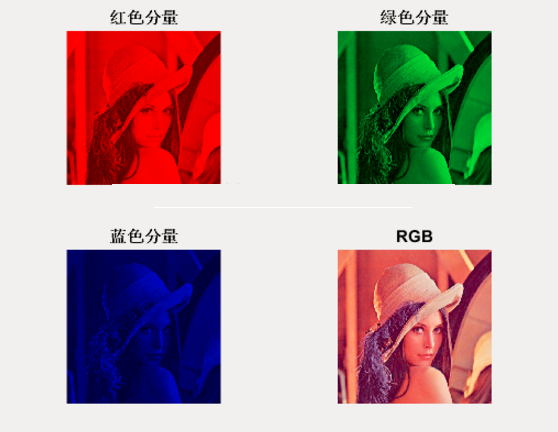‘这位同学，你说的简单，其实我对卷积层怎么实现特征提取完全不懂’

RGB格式大家自己谷歌吧，这也不多说了

Output =

（诶，我为什么要说我们）

W1output = 1*(-1) +1*1+1*0+0*(-1)+1*0+2*1+0*(-1)+1*1+2*(-1) =1

W2output = 2*1+2*0+1*1+1*1+0*1+0*2+0*1+0*0+1*1=5

W3output = 1*(-1)+1*(-1)+0*(-1)+0*2+0*(1)+0*2+1*0+1*1+0*1 = -1

Bias = 0

Final_output =W1output + W2output+W3output+bias= 1+5-1+0 = 5

（1）我们获得的更多更细致的特征信息，上面那个例子我们就可以获得更多的图像边缘信息

（1）一张图片的特征千千万，卷积核也有千千万，我们是怎样来确定卷积核的数值是可以提取我们想要的特征，或者说能提取使得最后分类正确的特征呢？

（2）卷积核里面的权值是如何更新的呢？

2018-0203现在回来看感觉这文章挖了些坑没讲清楚